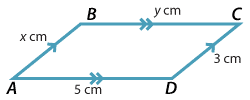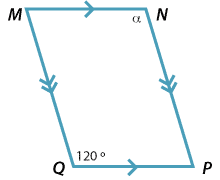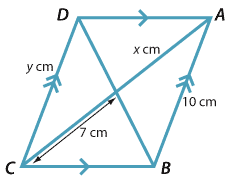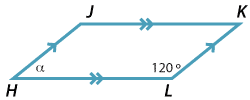### Parallelograms

#### Question 1

Use the properties of a parallelogram to find the values of $$x$$, $$y$$ and α in the diagrams below.

a i$$x$$ = cm $$y$$ = cmα = °Detailed description $$x$$ = cm $$y$$ = cmα = °